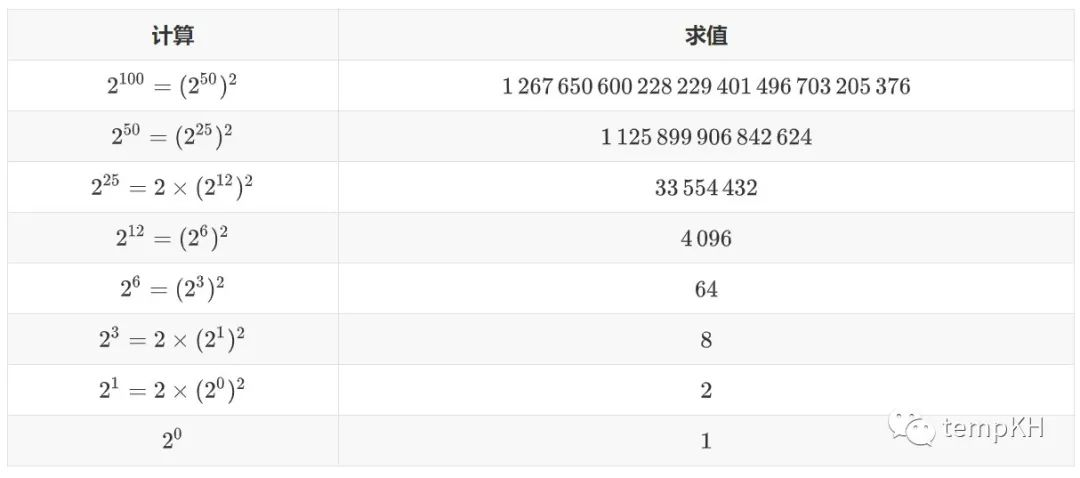# 如何快速算出一个数的n次方？

“幂”在许多地方常被用到，如 Hash 相关、费马小定理、进制转换等。function power(a, n):
if n = 0 then return 1
t := power(a, (n - n mod 2) / 2)
if n mod 2 = 1
then: return t^2 * a
else: return t^2


……

function power(a, n):
pow[0...log n], pow := 1
for i: 1 to inf
if (2^i > n) break
else pow[i] = pow[i - 1]^2
res := 1
for i: 1 to inf
if (2^i > n) break
else:
if (n bitand 2^i) res = res * pow[i]
return res
# n bitand 2^i 可理解为 n 在二进制表示下含 2^i 位

function power(a, n):
res := 1, p := a
while n > 0:
if (n bitand 1) then res = res * a
p = p^2, n = n >> 1
return res
# bitand 表示按二进制位与，>> 表示按二进制位右移（可理解为除以 2 下取整）。


function times(a, b, m):
if b = 0 then return 0
t := times(a, (b - b mod 2) / 2, m)
if b mod 2 = 1
then: return ((t + t) mod m + a mod m) mod m
else: return (t + t) mod m


function power(a, b, m):
res := 0
while b > 0:
if (b bitand 1) then res = (res + a) mod m
a = (a + a) mod m, b = b >> 1
return res
# bitand 表示按二进制位与，>> 表示按二进制位右移（可理解为除以 2 下取整）。


03-171万+
11-12401903-147万+
04-122万+
05-183720
05-103027
05-141033
01-039万+
06-012255
04-229237
06-28628
09-093171
01-121001
05-19666
03-206084
09-061899
05-045667
10-183971
04-11880点击重新获取扫码支付余额充值